# 前言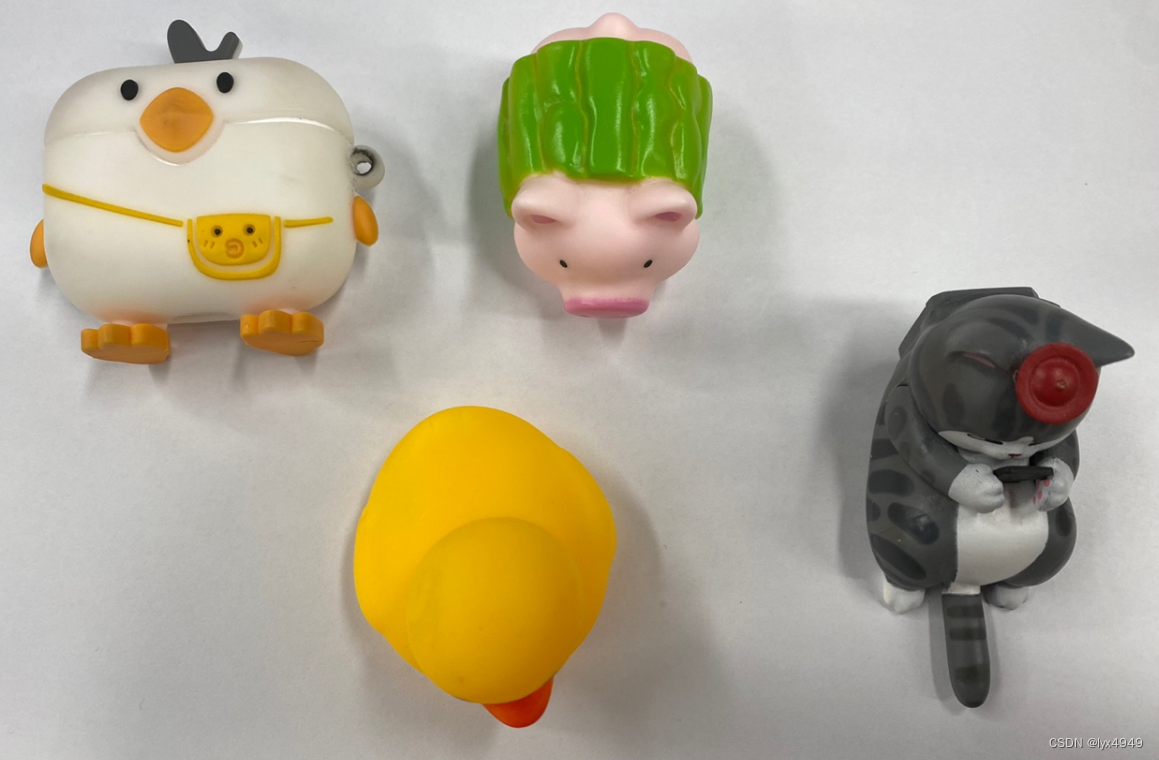# 一、项目内容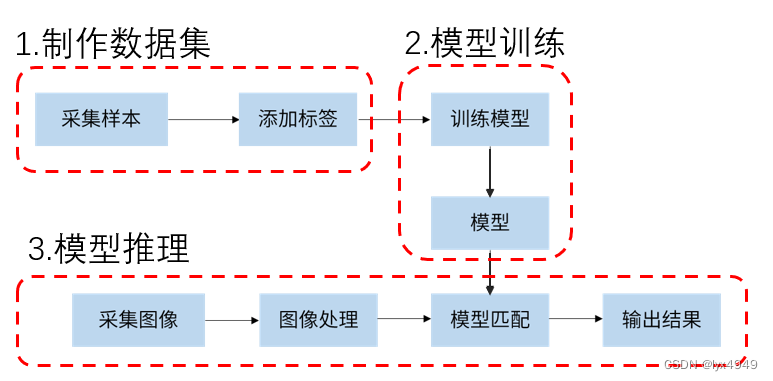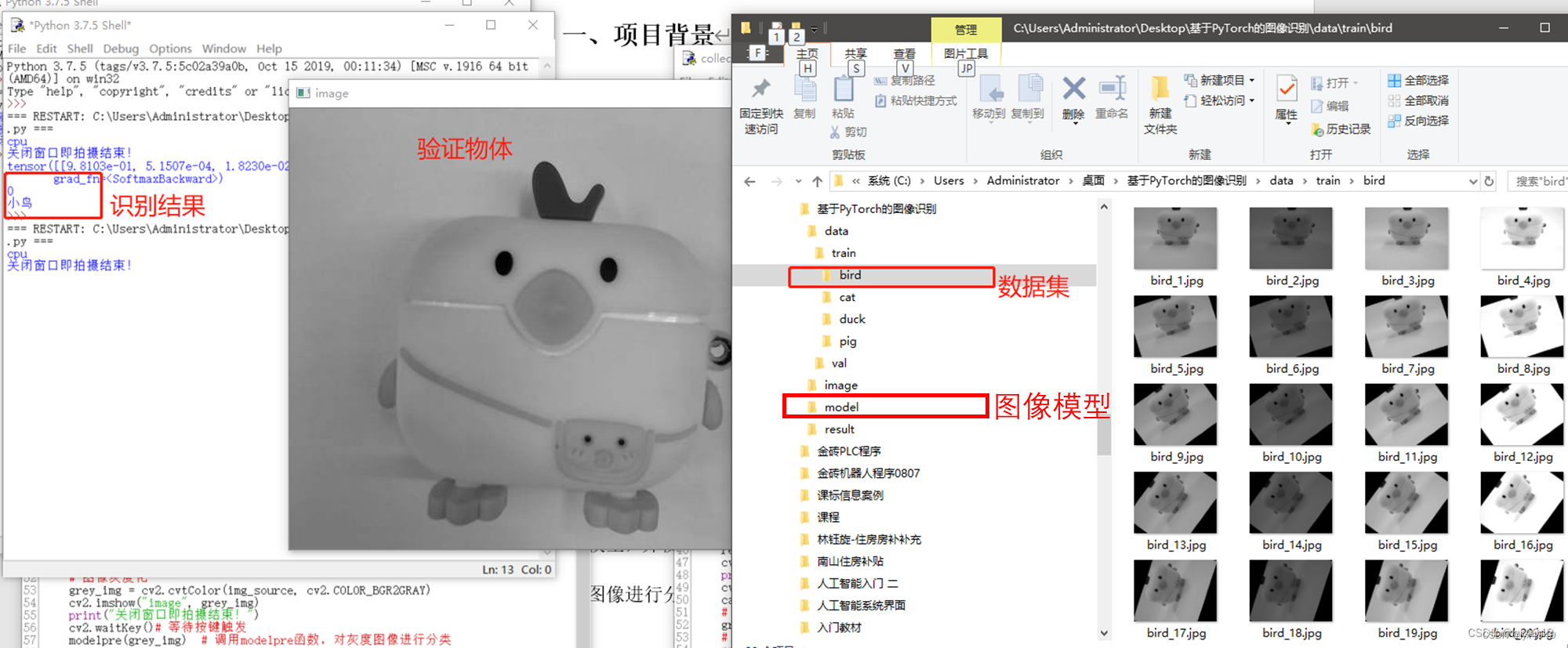# 二、知识链接

（此部分是关于Pytorch如何实现图像识别的流程介绍，可以直接跳到下一步）

• 图像采集及数据预处理

• 定义模型（训练模型过程）

• 定义损失函数（训练模型过程）

• 定义优化器（训练模型过程）

• 训练模型（训练模型过程）

• 模型推理

# 三、项目准备

1.摄像头：能够拍摄到图像的工具，建议外界摄像头能够拍摄到物体的图片。
2.如果没有外接摄像头，可以自己手动采集相关的物体图片（例如鸟、鸭、猪、猫）
3.可以看最后我上传的附件程序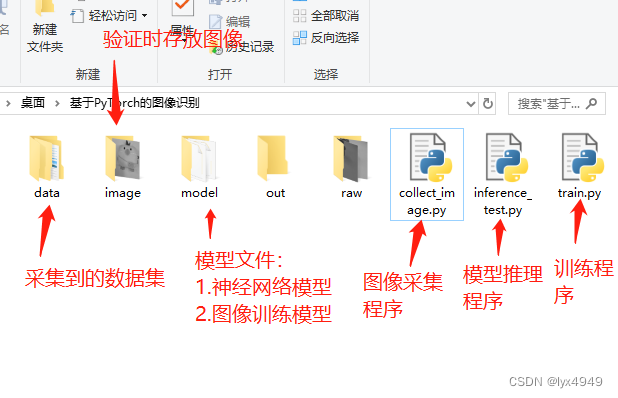# 四、项目实施

## 任务一：数据集准备

1.本程序分为2部分：①采集数据集 ②拍摄一张图像（等到训练好后使用）
① 采集数据集：使用外接摄像头拍摄物体的图片并生成4张曝光度不同的灰度图片存放到raw文件夹，然后把这4张图片分别旋转20次，每次18°
②拍摄一张图像此部分用于训练好模型之后使用

``````#collect_image.py程序
import cv2
import os
import numpy as np
#采集物体4张曝光度不同的图像，然后旋20次，每次18°
def Collect_Datasets():
# 创建文件夹
if not os.path.exists('raw'):  #原始4张曝光度图片存放路径
os.makedirs('raw')
if not os.path.exists('out'):  #旋转后生成图片的路径
os.makedirs('out')
image_name = input("请输入物体名称：")  #给拍摄物体命名
if not os.path.exists(image_name):
os.makedirs(f'data/train/{image_name}')  #把物体数据集存放到data/tarin文件夹
#采集物体4张曝光度不同的图像
# 调用摄像头拍摄1张图片
cap = cv2.VideoCapture(1)
cap.release()
# 灰度化
gray_frame = cv2.cvtColor(frame, cv2.COLOR_BGR2GRAY)
# 获取图像宽度和高度
height, width = gray_frame.shape
# 保存第一张灰度图片到raw文件夹
cv2.imwrite('raw/exposure_1.jpg', gray_frame)
# 生成其他3张曝光度不同的图片
for i in range(3):

#旋转图片
for i in range(20):
angle = i * 18  # 每次旋转18°
for j in range(4):
image_path = f'raw/exposure_{j+1}.jpg'  #给旋转的图片命名
#cv2.getRotationMatrix2D()函数用于获取图像旋转的变换矩阵
#获取一个变换矩阵 M，该矩阵可以将图像围绕 (width/2, height/2) 旋转 angle 度
M = cv2.getRotationMatrix2D((width/2, height/2), angle, 1)
rotated = cv2.warpAffine(img, M, (width, height))    #对图像进行旋转操作
out_path = f'data/train/{image_name}/{image_name}_{i*4+j+1}.jpg'  #图片存放路径，存放到以你为物体命的名的文件夹
cv2.imwrite(out_path, rotated)
#拍摄一张需要验证的物体图像，用于训练完验证时候使用
def Verify_Image():
print("拍摄一张图片验证数据集！")
cap = cv2.VideoCapture(1)
cv2.imshow("image", frame)
print("关闭窗口即拍摄结束！")
cv2.waitKey()# 等待按键触发
cap.release()
# 灰度化
gray_frame = cv2.cvtColor(frame, cv2.COLOR_BGR2GRAY)
# 保存灰度图片
cv2.imwrite('image/image.jpg', gray_frame)

if __name__ == "__main__":
collect_picture = input("请输入数字：1.采集数据集 2.采集一张验证:")
if collect_picture == "1":
Collect_Datasets()  #制作数据集
elif collect_picture == "2":
Verify_Image()  #验证时采集一张图片的时候调用

``````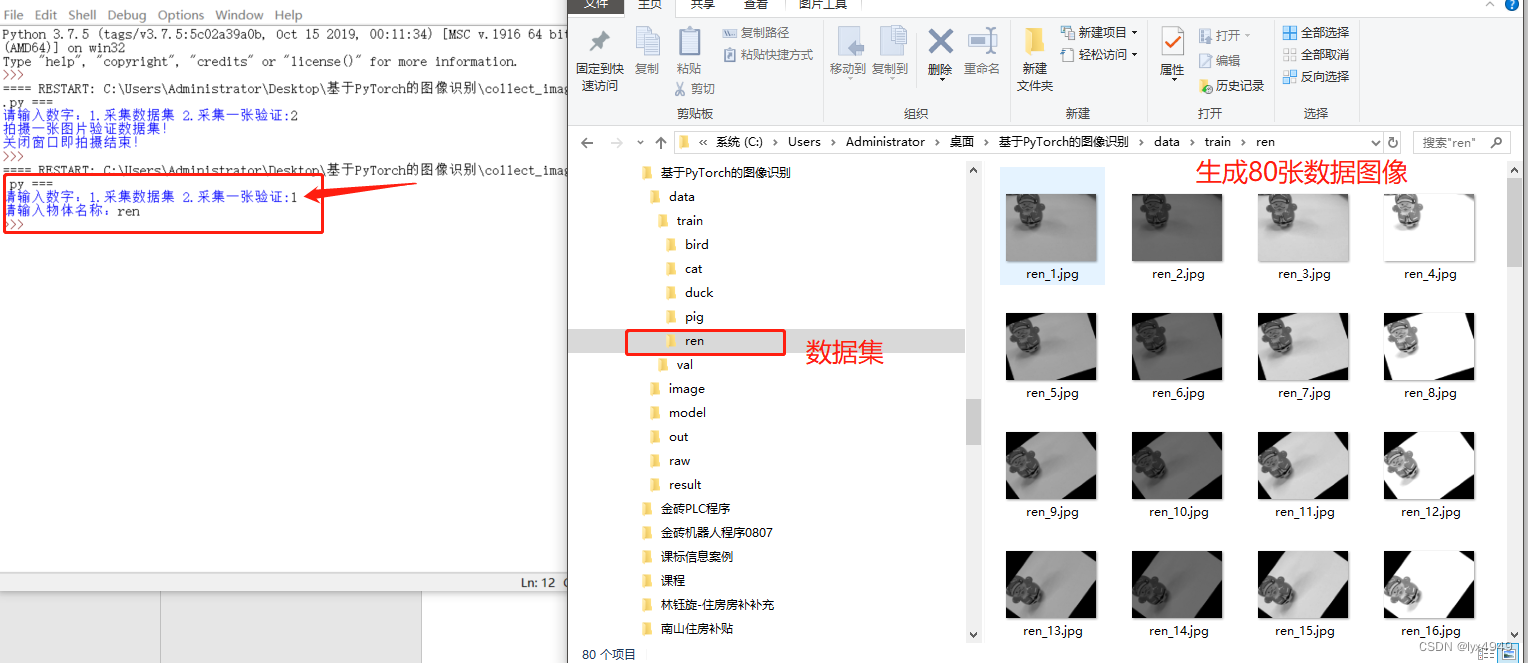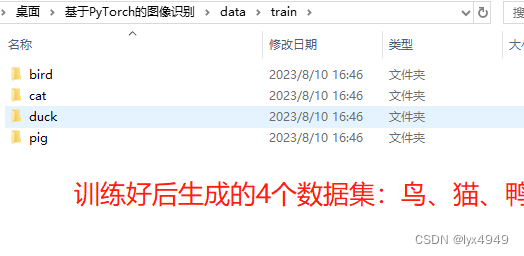## 任务二：模型训练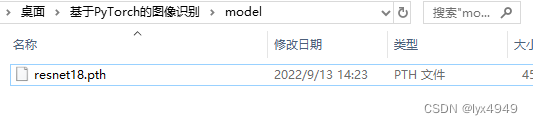``````#train.py程序
import os
import time
import torch
import torchvision
from torchvision import datasets, models, transforms
import matplotlib.pyplot as plt
import numpy as np
import copy
import glob
import shutil
import torch.nn as nn
import torch.optim as optim
from torch.optim import lr_scheduler

# 数据集存放路径
path = './data/'

# 遍历数据集
#将训练集数据中的数据随机抽取作为验证集数据，并将验证集数据复制到新建的文件夹中，以便于后续的模型训练和评估
for folder in os.listdir('./data/train'):
# 图片格式为.jpg或.png
jpg_files = glob.glob(os.path.join(path,"train", folder, "*.jpg"))
png_files = glob.glob(os.path.join(path,"train", folder, "*.png"))
files = jpg_files + png_files
# 统计训练集数据
num_of_img = len(files)
print("Total number of {} image is {}".format(folder, num_of_img))
# 从训练集里面抽取100%作为验证集,将训练集中的所有数据进行随机打乱，并计算出需要抽取的数据数量
shuffle = np.random.permutation(num_of_img)
percent = int(num_of_img * 1)
print("Select {} img as valid image".format(percent) )
# 新建val文件夹存放验证集数据
path_val = os.path.join(path,"val",folder)
if not os.path.exists(path_val):
os.makedirs(path_val)
# 把训练集里面抽取100%的数据复制到val文件夹
# shuffle()方法将序列的所有元素随机排序
for i in shuffle[:percent]:
print("copy file {} ing".format(files[i].split('\\')[-1]))
shutil.copy(files[i], path_val)

# 数据增强与变换
'''

'''
data_transforms = {
# 训练集
'train':transforms.Compose([
transforms.Resize((224,224)),   #调整输入图像的大小,以适应模型的输入尺寸
transforms.Grayscale(3),        #将输入图像转换为三通道的灰度图像，以便于在RGB模型上进行训练
transforms.RandomRotation(5),   #对输入图像随机进行旋转
transforms.RandomVerticalFlip(0.5),     #对输入图像进行垂直翻转，增加模型对镜像变换的鲁棒性
transforms.RandomHorizontalFlip(0.5),   #对输入图像进行水平翻转，增加模型对镜像变换的鲁棒性
transforms.ToTensor(),                  #将输入图像转换为PyTorch张量
transforms.Normalize([0.5,0.5,0.5], [0.5,0.5,0.5]) #对输入图像进行标准化处理
]),
# 测试集，同上
'val':transforms.Compose([
transforms.Resize((224,224)),
transforms.Grayscale(3),
transforms.RandomRotation(5),
transforms.RandomVerticalFlip(0.5),
transforms.RandomHorizontalFlip(0.5),
transforms.ToTensor(),
transforms.Normalize([0.5,0.5,0.5], [0.5,0.5,0.5])
])
}

# 加载数据
data_dir = './data/'
# 使用datasets.ImageFolder()方法加载数据集，并对训练集和验证集分别进行数据增强和变换操作
image_datasets = {x: datasets.ImageFolder(os.path.join(data_dir, x), data_transforms[x]) for x in ['train','val'] }
print(image_datasets)
batch_size=len(os.listdir('./data/train')),  #batch_size参数表示每个批次的样本数量
shuffle=True,  #shuffle参数表示是否对数据进行打乱
num_workers=0) for x in ['train', 'val'] } #num_workers参数表示数据加载的并行数
# 统计训练集和验证集的数据集大小
dataset_sizes = {x:len(image_datasets[x]) for x in ['train', 'val']}
print(dataset_sizes)
# 获取数据集的类别信息
class_names = image_datasets['train'].classes
print(class_names)

# 判断GPU是否可用,如果当前计算机支持GPU，则使用GPU作为计算设备；否则使用CPU作为计算设备
device = torch.device("cuda:0" if torch.cuda.is_available() else "cpu")
print(device)

# 通用训练模型函数,参数说明：模型、损失函数、优化器、学习率调度器、可选的训练轮数
'''

'''
def train_model(model, criterion, optimizer, scheduler, num_epochs=25):
since = time.time() # 返回当前时间的时间戳
# deepcopy为深拷贝,即创建一个新的对象，完全复制原始对象及其所有子对象。
# model.state_dict是PyTorch中的一个方法，返回一个字典对象，将模型的所有参数映射到它们的张量值
best_model_wts = copy.deepcopy(model.state_dict())
best_acc = 0.0
# 显示当前的训练进度
for epoch in range(num_epochs):
print('Epoch {}/{}'.format(epoch, num_epochs - 1)) # 输出训练进度
print('-' * 10)
# 每个epoch都有一个训练和验证阶段
for phase in ['train', 'val']:
if phase == 'train':
# PyTorch中用于更新优化器的学习率
scheduler.step()
model.train()  # 设置模型的工作模式：训练模式，模型会更新参数
else:
model.eval()   # 设置模型的工作模式：评估模式，模型不会更新参数
running_loss = 0.0  # 用于记录当前epoch的累计损失
running_corrects = 0 # 用于记录当前正确分类的数量
# 迭代数据
# GPU加速,inputs、labels用于将输入数据和标签数据移动到指定的设备上，例如CPU或GPU
inputs = inputs.to(device)
labels = labels.to(device)
# 清空梯度，在每次优化前都需要进行此操作
outputs = model(inputs)
_, preds = torch.max(outputs, 1) # 返回输入张量在指定维度上的最大值及其对应的索引
loss = criterion(outputs, labels)
# 后向 + 仅在训练阶段进行优化
if phase == 'train':
# 反向传播：根据模型的参数计算loss的梯度
loss.backward()
# 调用Optimizer的step函数使它所有参数更新
optimizer.step()
running_loss += loss.item() * inputs.size(0) # 获取损失值和输入数据的数量
running_corrects += torch.sum(preds == labels.data) # 记录当前epoch中正确分类的数量
# dataset_sizes[phase]：该变量用于获取数据集在当前阶段中的大小
epoch_loss = running_loss / dataset_sizes[phase]  #当前epoch的平均损失
epoch_acc = running_corrects.double() / dataset_sizes[phase]  # 当前epoch的准确率
# 打印当前epoch的损失和准确率，以实时监控模型的训练状态
print('{} Loss: {:.4f} Acc: {:.4f}'.format(
phase, epoch_loss, epoch_acc)) # 前阶段的名称、当前epoch的平均损失、当前epoch的准确率
if phase == 'val' and epoch_acc > best_acc:  #更新最佳模型参数
best_acc = epoch_acc
best_model_wts = copy.deepcopy(model.state_dict())
print()
time_elapsed = time.time() - since
# 输出整个训练过程的时间
print('Training complete in {:.0f}m {:.0f}s'.format(
time_elapsed // 60, time_elapsed % 60))
# 用于输出最佳验证准确率,best_acc是训练过程中被更新为模型在验证集上的最佳准确率
print('Best val Acc: {:4f}'.format(best_acc))
# 用于加载模型的状态字典，即将最佳模型的参数设置为给定的参数字典
return model

# 主程序入口使用ResNet-18模型对图像进行分类，并训练模型并保存模型参数。
if __name__ == "__main__":
# 用于初始化ResNet-18 模型
model_ft = torchvision.models.resnet18(pretrained=False)
# 加载resnet18网络参数
# 提取fc层中固定的参数
num_ftrs = model_ft.fc.in_features
# 重写全连接层的分类
model_ft.fc = nn.Linear(num_ftrs, len(os.listdir('./data/train')))
model_ft = model_ft.to(device)
# 这里使用分类交叉熵Cross-Entropy作为损失函数，动量SGD作为优化器
criterion = nn.CrossEntropyLoss()
# 初始化优化器
optimizer_ft = optim.SGD(model_ft.parameters(), lr=0.001, momentum=0.9)
# 每7个epochs衰减LR通过设置gamma = 0.1
exp_lr_scheduler = lr_scheduler.StepLR(optimizer_ft, step_size=7, gamma=0.1)
#调用通用训练模型的函数，返回训练得到的最佳模型
'''
下面调用了定义好的模型的训练函数。最后一个参数num_epochs用于指定训练模型的轮数，训练模型的轮数是一个重要的参数，
它决定了模型训练的时间和精度。训练轮数太短可能会导致模型欠拟合，训练轮数太长则可能会导致模型过拟合。
因此，在选择训练轮数时需要进行适当的调整和优化，以得到最佳的模型性能。
在这个例子中，由于数据集较小，所以训练轮数设置为 2 轮，在实际应用中，
需要根据数据集的大小、复杂度和模型的性能等因素进行调整，以便得到更为准确和实用的模型。
'''
model_ft = train_model(model_ft, criterion, optimizer_ft, exp_lr_scheduler, num_epochs=2)
# 保存模型
torch.save(model_ft.state_dict(), './model/dobot20230810.pkl')

``````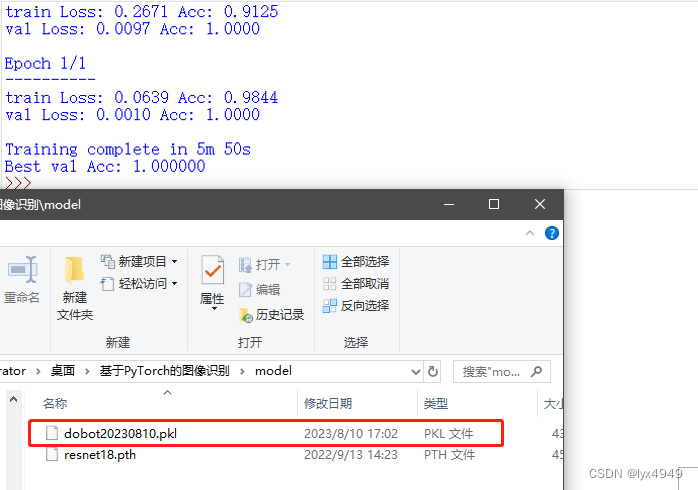## 任务三：模型推理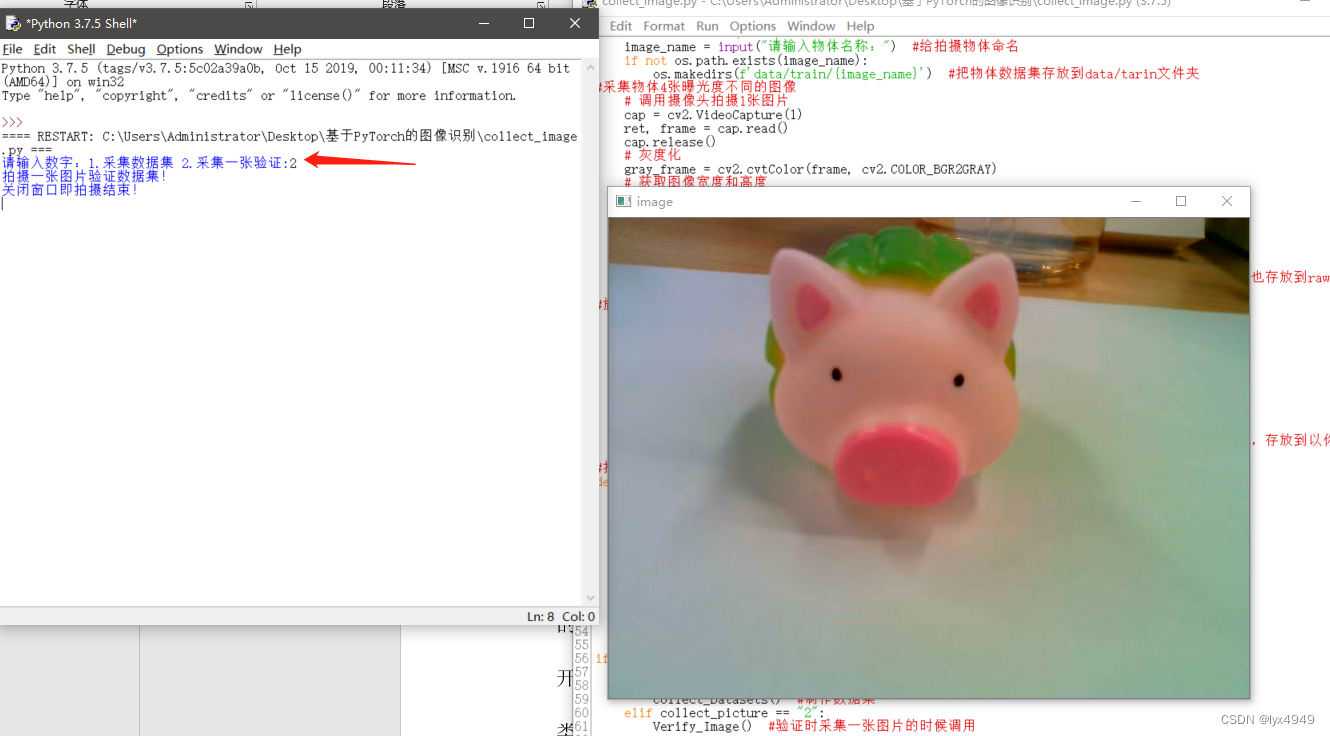``````#inference_test.py程序
import cv2
from PIL import Image
import torch
import torchvision
from torchvision import datasets, models, transforms
import torch.nn.functional as F
import torch.nn as nn

tsfrm = transforms.Compose([
transforms.Grayscale(3),        # 将图像转换为灰度图像，通道数为3
transforms.Resize((224, 224)),  # 将图像变换为指定大小
transforms.ToTensor(),          # 将PIL图像转换为tensor数据
transforms.Normalize([0.5, 0.5, 0.5], [0.5, 0.5, 0.5])      # 将PIL图像转换为tensor数据
])

# 0.水果 1.蔬菜 2.服装 3.零食 (顺序需要按照训练集中的顺序)
classes = ('小鸟','小猫','小鸭','小猪')

# 判断GPU是否可用，有cpu和cuda两种，这里的cuda就是gpu
device = torch.device("cuda:0" if torch.cuda.is_available() else "cpu")
print(device)

# 模型推理
def modelpre(grey_img):
model_eval = models.resnet18(pretrained=False)  # 加载ResNet18模型
num_ftrs = model_eval.fc.in_features   # 获取默认的全连接层的输入维度
model_eval.fc = nn.Linear(num_ftrs, 4)  # 替换模型的全连接层，使其输出4个分类
# 加载已经训练好的模型权重
# 在推理前，务必调用model.eval()去设置dropout和batch normalization层为评估模式
model_eval.eval()
# 将OpenCV图像转换为PIL图像，并进行预处理
image = Image.fromarray(cv2.cvtColor(grey_img, cv2.COLOR_GRAY2RGB))
# PIL图像数据转换为tensor数据，并归一化
img = tsfrm(image)
# 图像增加1维[batch_size,通道,高,宽]
img = img.unsqueeze(0)
# 进行模型推理，得到分类结果
output = model_eval(img)
# 计算每个分类的概率 prob是4个分类的概率
prob = F.softmax(output, dim=1)
print(prob) # tensor([[0.0360, 0.0061, 0.9529, 0.0050]], grad_fn=<SoftmaxBackward>)
# 找出最大概率的分类.torch.max() 函数会返回输入张量中最大值和最大值的索引
value, predicted = torch.max(output.data, 1) # value模型预测出来的最大概率值
label = predicted.numpy()   # 将Tensor转换为numpy数组
print(label)  # 打印出标签的索引
pred_class = classes[predicted.item()]  # 提取最佳分类的标签
print(pred_class)

if __name__ == "__main__":
# 图像灰度化
grey_img = cv2.cvtColor(img_source, cv2.COLOR_BGR2GRAY)
cv2.imshow("image", grey_img)
print("关闭窗口即拍摄结束！")
cv2.waitKey()# 等待按键触发
modelpre(grey_img)  # 调用modelpre函数，对灰度图像进行分类

``````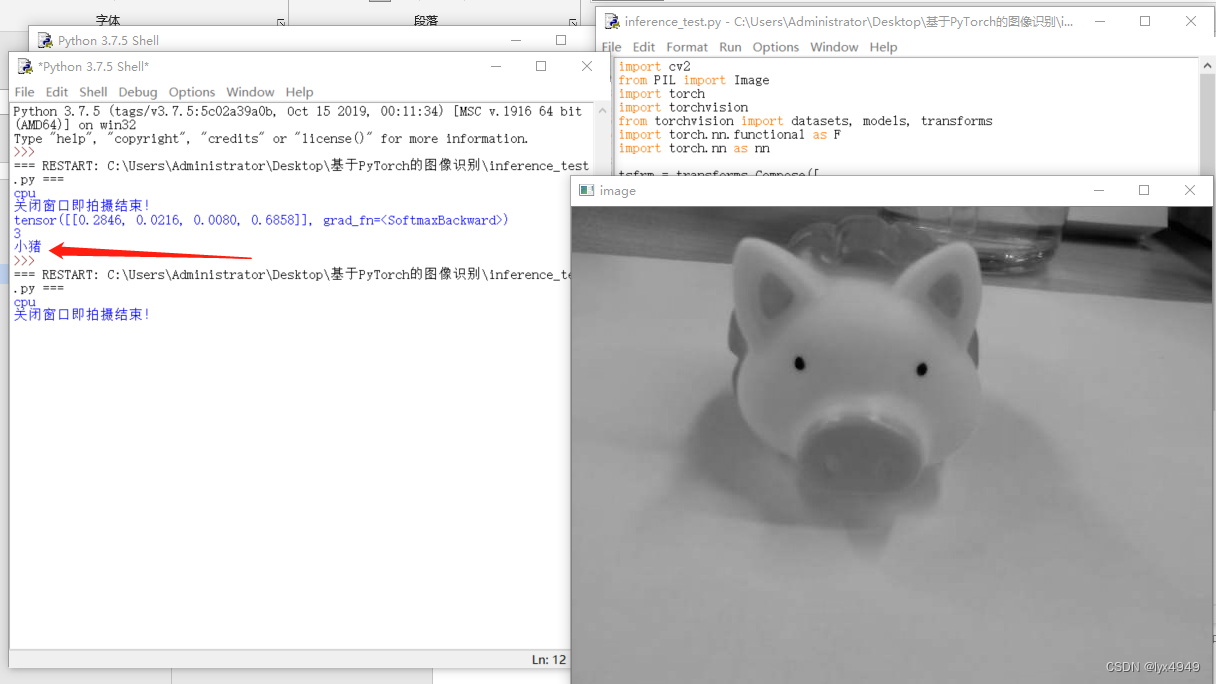# 评论

### 评论列表

##### 微信小程序##### QQ小程序